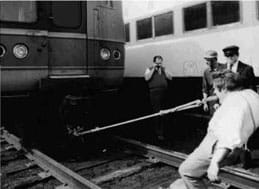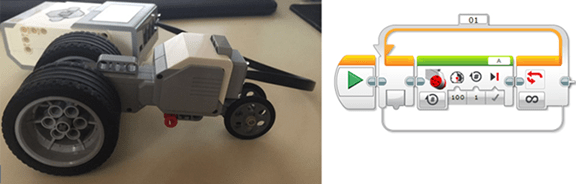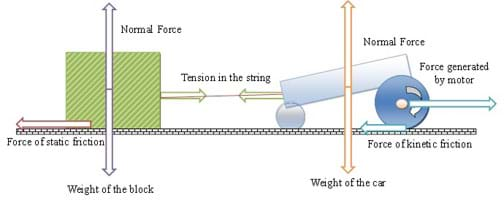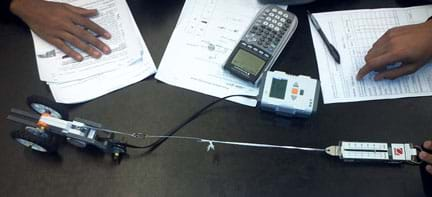# Hands-on ActivityHow to Pull Something Heavy

(0 Ratings)

### Quick Look

Time Required: 1 hours 15 minutes

Expendable Cost/Group: US \$0.00

This activity requires the use of non-expendable (and highly reusable) LEGO® MINDSTORMS® robot kits, one per group (\$300 each); see the Materials List for details.

Group Size: 3

Activity Dependency: None

Subject Areas: Physics, Science and Technology

NGSS Performance Expectations:### Summary

Students measure and analyze forces that act on vehicles pulling heavy objects while moving at a constant speed on a frictional surface. They study how the cars interact with their environments through forces, and discover which parameters in the design of the cars and environments could be altered to improve vehicles' pulling power. This LEGO® MINDSTORMS® based activity is geared towards, but not limited to, physics students.
This engineering curriculum aligns to Next Generation Science Standards (NGSS).

### Engineering Connection

Understanding forces and how they interact with their environments has enabled engineers to create vehicles with amazing pulling power, such as tow trucks, fork lifts and tractors, and even snowmobiles and tugboats. One early stage of the engineering design process involves analysis of a project design using conceptual physics and mathematical models, which are used to develop a description of how objects interact with one another. Through these models, engineers are able to better problem-solve, understand, troubleshoot and improve the design of their projects, devices or products.

### Learning Objectives

After this activity, students should be able to:

• Identify forces and draw a free body diagram of a vehicle pulling on a heavy object.
• Describe the connection between force of friction and weight of the object.
• Explain the difference between pulling force and force generated by the motor.

### Educational Standards Each TeachEngineering lesson or activity is correlated to one or more K-12 science, technology, engineering or math (STEM) educational standards. All 100,000+ K-12 STEM standards covered in TeachEngineering are collected, maintained and packaged by the Achievement Standards Network (ASN), a project of D2L (www.achievementstandards.org). In the ASN, standards are hierarchically structured: first by source; e.g., by state; within source by type; e.g., science or mathematics; within type by subtype, then by grade, etc.

###### NGSS: Next Generation Science Standards - Science
NGSS Performance Expectation

HS-PS2-1. Analyze data to support the claim that Newton's second law of motion describes the mathematical relationship among the net force on a macroscopic object, its mass, and its acceleration. (Grades 9 - 12)

Do you agree with this alignment?

Click to view other curriculum aligned to this Performance Expectation
This activity focuses on the following Three Dimensional Learning aspects of NGSS:
Science & Engineering Practices Disciplinary Core Ideas Crosscutting Concepts
Analyze data using tools, technologies, and/or models (e.g., computational, mathematical) in order to make valid and reliable scientific claims or determine an optimal design solution.

Alignment agreement:

Theories and laws provide explanations in science.

Alignment agreement:

Laws are statements or descriptions of the relationships among observable phenomena.

Alignment agreement:

Newton's second law accurately predicts changes in the motion of macroscopic objects.

Alignment agreement:

Attraction and repulsion between electric charges at the atomic scale explain the structure, properties, and transformations of matter, as well as the contact forces between material objects.

Alignment agreement:

Empirical evidence is required to differentiate between cause and correlation and make claims about specific causes and effects.

Alignment agreement:

###### Common Core State Standards - Math
• Reason abstractly and quantitatively. (Grades K - 12) More Details

Do you agree with this alignment?

• (+) Solve problems involving velocity and other quantities that can be represented by vectors. (Grades 9 - 12) More Details

Do you agree with this alignment?

• Use units as a way to understand problems and to guide the solution of multi-step problems; choose and interpret units consistently in formulas; choose and interpret the scale and the origin in graphs and data displays. (Grades 9 - 12) More Details

Do you agree with this alignment?

• Solve linear equations and inequalities in one variable, including equations with coefficients represented by letters. (Grades 9 - 12) More Details

Do you agree with this alignment?

###### International Technology and Engineering Educators Association - Technology
• Students will develop an understanding of the relationships among technologies and the connections between technology and other fields of study. (Grades K - 12) More Details

Do you agree with this alignment?

• Students will develop an understanding of the attributes of design. (Grades K - 12) More Details

Do you agree with this alignment?

###### New York - Math
• Reason abstractly and quantitatively. (Grades Pre-K - 12) More Details

Do you agree with this alignment?

• Solve linear equations and inequalities in one variable, including equations with coefficients represented by letters. (Grades 9 - 12) More Details

Do you agree with this alignment?

• Use units as a way to understand problems and to guide the solution of multi-step problems; choose and interpret units consistently in formulas; choose and interpret the scale and the origin in graphs and data displays. (Grades 9 - 12) More Details

Do you agree with this alignment?

• (+) Solve problems involving velocity and other quantities that can be represented by vectors. (Grades 9 - 12) More Details

Do you agree with this alignment?

###### New York - Science
• Analyze data to support the claim that Newton's Second Law of Motion describes the mathematical relationship among the net force on a macroscopic object, its mass, and its acceleration. (Grades 9 - 12) More Details

Do you agree with this alignment?

Suggest an alignment not listed above

### Materials List

For the teacher's introductory presentation and activity preparation:

Each group needs:

Note: This activity can also be conducted with the older (and no longer sold) LEGO MINDSTORMS NXT set instead of EV3; see below for those supplies:

• NXT, motor, and building blocks from a LEGO MINDSTORMS NXT robot kit (instructions to build and program a basic robot are included with the kit; LEGO MINDSTORMS NXT robot, such as the NXT Base Set
• LEGO MINDSTORMS program: Move Robot Program (rbt)

### More Curriculum Like This

Upper Elementary LessonPowerful Pulleys

Students learn how a pulley can be used to change the direction of applied forces and move/lift extremely heavy objects, and the powerful mechanical advantages of using a multiple-pulley system. Students perform a simple demonstration to see the mechanical advantage of using a pulley, and they ident...

Middle School LessonBridging the Gaps

Students are presented with a brief history of bridges as they learn about the three main bridge types: beam, arch and suspension. They are introduced to two natural forces — tension and compression — common to all bridges and structures.

### Pre-Req Knowledge

Students should have an understanding of forces and motion, as well as vector notation.

### Introduction/Motivation

(Prepare to show the class the attached eight-slide How to Pull Something Heavy Presentation, a PowerPoint file.)

What does it take to pull something heavy? What are the factors at play if you (or a machine) are trying to tug at a heavy object and draw it towards yourself? Let's take a look at an event that is pictured here (show Figure 1, or slide #3).Figure 1. In 1974, John Massis pulled two railroad passenger cars, weighing 80 tons, with a thick rope held in his teeth.copyrightCopyright © AP/Wide World Photos, Library of Congress in Halliday, Resnick and Walker, Fundamentals of Physics. 6th edition, John Wiley & Sons, 2001

(Read the following text to the students as they look at the image.) "On April 4, 1974, John Massis of Belgium managed to move two passenger cars belonging to New York's Long Island Railroad. He did so by clamping his teeth down on a bit that was attached to the cars with a rope and then leaning backward while pressing his feet against the railway ties. The cars together weighed about 80 tons. Did Massis have to pull with superhuman force to accelerate them?" (Halliday, 2001) How did he do it?

The phenomenon of Massis' achievement is of interest to engineers who work in fields of biomechanics, mechatronics and robotics, material science and engineering—just to name of few. In all these fields, engineers are interested in two types of "how" questions about Massis' achievement:

• How did Massis take advantage of the force of friction, gravity and his own power to pull 80 tons?
• How can we engineer a robot or a vehicle that can mimic Massis in pulling such a massive weight?

To discover the answers to these questions, we need to learn what is called "dynamics."

Dynamics is a study of causes of motion, namely forces that cause objects to start or to continue moving. To engineer and create mechanical advantage using forces, we must understand what stationary and moving objects experience when they interact with particular environments. Specifically, we need to understand that forces have magnitudes and directions, and that they interact with each other. The interaction of forces can be explained by the three laws of motion described by Isaac Newton. For example, Newton's third law of motion (also known as the law of interaction) tells us that forces always occur in pairs. (Tipler, 1995).

In today's hands-on activity, we will use a LEGO-based car to mimic Massis' record-breaking achievement. In particular, we will study how this car is interacting with its environment by studying forces that act on it and we will discover which parameters we can manipulate to enable a car to have more pulling power.

### Procedure

Before the Activity

• Gather materials and make copies of the How to Pull Something Heavy Worksheet and the Coefficient of Friction Table.
• Build one or more LEGO MINDSTORMS-based vehicles, as shown in Figure 2, following the LEGO Digital Designer Instructions for the Battle Bot Robot (lxf), which requires the LEGO Digital Designer application to open and provides a step-by-step guide on how to put LEGO pieces together to build a vehicle). Build enough for one per group. Make sure each robot is functioning; see the Troubleshooting Tips section.
• Download the LEGO MINDSTORMS Move Robot Program (rbt) onto the EV3 brick. The program, to move the car during the experiment, is illustrated in Figure 2.
• Provide a 5-Newtons spring scale and weight scale for each team.
• Prepare a computer and LCD projector to show the PowerPoint presentation.Figure 2. The LEGO-based car for the activity uses one motor and one EV3 brick (left), and the Move Robot Program (ev3) drives the car during the experiment (right).copyrightCopyright © 2011 Irina Igel, AMPS GK-12 Program, Polytechnic Institute of NYU

With the Students

1. With the PowerPoint presentation displayed to the class, facilitate a class discussion with students about vehicles that are used to pull heavy objects. Ask them to give examples of such vehicles and the circumstances of their use. See slide #2 for examples: fork lift, tractor, tow truck.
2. Discuss with students Massis' record-breaking challenge, as described in the Introduction/Motivation section (and shown on slide #3). Use this discussion as a transition to explain that the goal of the activity is to analyze how a moving vehicle interacts with an environment through forces.
3. Distribute worksheets to the students. Give them two or three minutes to complete the "Do now" section. On the worksheet, students analyze forces acting on a car-object system; see Figure 3. Note that the system is in equilibrium, that is, the green block is assumed to be heavy such that a car cannot move it from its position (also see slide #4).Figure 3. An illustration of a car pulling on a heavy object and its corresponding free body diagram.copyrightCopyright © 2011 Irina Igel, AMPS GK-12 Program, Polytechnic Institute of NYU
4. The slides in the presentation provide a free body diagram for the "Do now" problem, the derivation of the dynamics of the problem, and analysis of dynamics in a case in which a car is able to pull the block. In this case, we derive an equation of a pulling or tugging force of the car, that is:

Fpull = Fmotor – FwheelFriction = Fmotor – μkinetic * Wcar

Pulling force is the tugging force that the block experiences when the car is pulling on it. If the weight of the car, material of the tires and the EV3 battery power level remain constant for a particular car, the pulling force is constant no matter what object the car is trying to pull. Hence, if we know the pulling force of a car, we can determine if it will be able to pull a static object that needs to be moved. Explain to students that during this activity they will measure the pulling force of the car by having it tug on a spring scale.

1. Start the hands-on portion of the activity:
• Divide the class into groups of three students each.
• Distribute the equipment necessary for each group.
• Ask students to turn on the EV3 and test run the Move Robot Program (ev3) on the LEGO car to familiarize themselves with the set-up.
• From previous knowledge and information conveyed during the presentation, expect students to be able to figure out which equation to use to derive the pulling force of the LEGO car. The worksheet also provides some guidance in the derivation of the pulling force.
1. Ask students to measure the mass of the LEGO car in kg. Note: Encourage students to write units next to their measurements. Have students calculate the weight and the normal force of the LEGO car, that is: Wcar = mcar * Gearth and Fnormal = Wcar. Challenge students to be inquisitive about the origin of these forces. For example, ask them:
• Where is the normal force coming from? (Answer: The floor is reacting to the force of the weight of the car.)
• How does Newton's third law explain the phenomenon? (Answer: The normal force magnitude is equal to the magnitude and opposite in direction of the force of gravity.)
1. Provide students with coefficients of static and kinetic friction between rubber tires and other materials; refer to the coefficient of friction table. Let students choose the surface on which they prefer to run their experiments. Have students look up kinetic coefficient of friction, μkinetic, between the rubber of the wheels and the chosen surface. Encourage students to observe whether a car slows down when it is interacting with the surface.
2. Have students calculate the force of friction experienced by the moving car on the chosen surface, that is, Ffriction = μkinetic * Fnormal. Encourage students to discuss how the force of friction, Ffriction, is related to the weight, Wcar, of the car, that is, Ffriction = μkinetic * Fnormal = μkinetic * Wcar.
3. Connect the Newton spring scale to the LEGO car and ask students to measure the pulling force (see Figure 4).Figure 4. Students take measurements of the pulling force of the LEGO car.copyrightCopyright © 2011 Irina Igel, AMPS GK-12 Program, Polytechnic Institute of NYU
4. Have students derive the relation between pulling force, force generated by the motor, Fmotor, and the force of friction, Ffriction, that is, Fpull = Fmotor – Ffriction.
5. Finally, have students calculate the force generated by the motor, Fmotor.
6. Lead a class discussion to compare results and review the concepts. Ask students the Investigating Questions. Collect the worksheets for grading. If time permits, ask students to draw free body diagrams of the examples of everyday situations that exhibit extreme pulling power (from the ideas and their answers to the last Investigating Question).
7. Conclude by assigning students the homework, as described in the Assessment section.

### Vocabulary/Definitions

coefficient of friction: A dimensionless quantity whose value depends on the material properties of two bodies placed in contact with one another. (Halliday, 2001) The coefficient relates the amount of friction force between two surfaces to the force holding the two surfaces together.

dynamics: The study of causes of motion, namely forces that cause objects to start or to continue moving.

force: An influence on an object that causes the object to change its velocity, that is, to accelerate. (Tipler, 1995)

force of friction: A force that occurs between two surfaces that are pressed closely together and are in motion relative to one another. In most situations, the force of friction is opposite the direction of motion.

free body diagram: A stripped-down diagram in which only one body is considered. That body is represented by a sketch or simply a dot. The external forces on the body are drawn, and a coordinate system is superimposed, oriented so as to simplify the solution. (Halliday, 2001)

kinetic friction: A force of friction that results when the friction between two surfaces is less than the force of motion.

Newton's second law of motion: The net force on a body is equal to the product of the body's mass and the acceleration of the body, that is, F = ma. (Halliday, 2001)

Newton's third law of motion: When two bodies interact, the forces on the bodies from each other are always equal in magnitude and opposite in direction. (Halliday, 2001)

parameter: A physical property whose value determines the behavior of the system.

static friction: A force of friction that results when the friction between two surfaces is greater or equal to the force of motion.

vector: A mathematical tool to represent quantities that have direction and magnitude. Vector is graphically represented by an arrow.

### Assessment

Pre-Activity Assessment

Worksheet: Have students complete the "Do now" section on the How to Pull Something Heavy Worksheet, review their answers to gauge their understanding of the concepts, including drawing of free body diagrams.

Activity Embedded Assessment

Worksheet: Have students perform the lab, a force analysis of a moving vehicle, and complete the "Data collection" section in the How to Pull Something Heavy Worksheet. Review their answers to evaluate their comprehension of the material.

Post-Activity Assessment

Homework: Assign students to complete the How to Pull Something Heavy Homework. Review their answers to gauge their mastery of the concepts.

### Investigating Questions

Keeping in mind the definition of a pulling force, why are light-weight race cars fast? (Answer: A car with less weight encounters less kinetic friction; hence, it is able to go faster. However, a car with too little weight is subject to wheel slip, hence, good design is required.)

Keeping in mind the definition of a pulling force, why are heavy-weight tractors slow? (Answer: A car with more weight experiences more resistance to its motion.)

What everyday situations can you think of that exhibit extreme pulling power? What situations require you to tug at a heavy object, drawing it towards yourself? What are the forces and environmental conditions present in these situations, for which engineers had to consider in their designs? (Possible answers: A truck pulling another car or truck out of a ditch or a snow bank. A tugboat hauling a barge down a river. A truck towing an RV or a boat. Your younger sister pulling the leash of your big dog to get it away from another dog [or the dog pulls her towards another dog!]. A locomotive pulling a train. Aircraft tug vehicles. A mover pulling a piano into your living room. A ship pulling an anchor off the sea floor. A rescue crew pulling out an elephant trapped in dried mud or a whale stuck on the beach. Competitors in a tug of war contest.)

### Troubleshooting Tips

Run each LEGO-based car experiment set-up before starting the activity to make sure that each group gets a functioning robot. When test-running a robot, make sure it does not slip during tugging. If the robot slips, add more weight to it.

### Activity Extensions

Conduct the Tug of War Battle Bots activity to introduce students to the concepts of torque, power, friction and gear ratios. Teams modify two robotic LEGO vehicles by changing their gear ratios, wheel sizes, weight, and engine power, while staying within a limit of points to spend on modifications. The robots face each other on a track with a string attaching one to the other. The winning robot, the one with the best adjustments, pulls the other across the line.

To further reinforce the concept of Ffriction = μkinetic * Wcar and understand traction, ask student to make another measurement of pulling force such that a heavy object (for example, a cell phone) is placed on the top of the LEGO-based car design. Here, traction is the maximum frictional force that can be produced between wheels and frictional surface without slipping.

### Activity Scaling

• For lower grades, skip the derivation of the Fpull and Fmotor and focus on the hands-on experimental portion of the activity. Ask students to measure the force of pull and forces of friction, and answer the "Analyze" question on the worksheet.

© 2013 by Regents of the University of Colorado; original © 2011 Polytechnic Institute of New York University

Irina Igel

### Supporting Program

AMPS GK-12 Program, Polytechnic Institute of New York University

### Acknowledgements

This activity was developed by the Applying Mechatronics to Promote Science (AMPS) Program funded by National Science Foundation GK-12 grant no. 0741714. However, these contents do not necessarily represent the policies of the NSF, and you should not assume endorsement by the federal government.

Additional support was provided by the Central Brooklyn STEM Initiative (CBSI), funded by six philanthropic organizations.